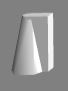# Rotating Around Axis

## How To Rotate Around an Axis About a Point

Rotation needs two things specifying, they are an axis and a center of rotation with the axis passing through the center of rotation. An axis is defined by a direction vector and the center of rotation a position vector. In Babylon.js when a mesh is created the center of rotation defaults to the the local origin of the mesh which is the mesh's position. Using rotation the axis is specified through the Euler angles alpha, beta, gamma, and using rotationQuaternion and rotate it is specified explicitly.

There are three ways to change a center of rotation different to the local origin, these are using a TransformNode, a parent and setting a pivot.

Together an axis and a center of rotation define a straight line in space and in the following animated playgrounds this line is shown in white. The asymmetric pilot mesh is used to demonstrate the rotation.The Pilot

In each of the following examples there is

• a center of rotation at CoR_At;
• a marker mesh for the center of rotation, a small sphere positioned at CoR_At;
• an axis to rotate around
• the pilot mesh at a starting position relative to the center of rotation, pilotStart.

## How to Use TransformNode as a Center of Rotation

A TransformNode is an object that is not rendered but can be used as a center of rotation (in fact the centre of any transformation). This can decrease memory usage and increase rendering speed.

The TransformNode is used as a pivot point by parenting it to the pilot and rotating it.

var pivot = new BABYLON.TransformNode("root");pivot.position = CoR_At;
pilot.parent = pivot;pilot.position = pilotStart;
pivot.rotate(axis, angle, BABYLON.Space.WORLD);

In these playgrounds a sphere is created only to show the position of the TransformNode.

Rotating TransformNode Rotating Mesh Moving TransformNode along Axis Rotating Mesh Moving TransformNode

## How To Use a Parent as Center of Rotation

The sphere is parented to the pilot, the pilot positioned and the sphere rotated.

sphere.position = CoR_At;
pilot.parent = sphere;pilot.position = pilotStart;
sphere.rotate(axis, angle, BABYLON.Space.WORLD);

Note: Any movement of the sphere will result in the pilot being moved.

Rotating Parent

## How To Use a Pivot as a Center of Rotation

var CoR_At = new BABYLON.Vector3(1, 3, 2);var pilotStart = new BABYLON.Vector3(3, 6, 6);
pilot.position = pilotStart;
var pivotTranslate = pilotStart.subtract(CoR_At);pilot.setPivotMatrix(BABYLON.Matrix.Translation(pivotTranslate.x, pivotTranslate.y, pivotTranslate.z));
/*-------------------Rotation Animation--------------------*/var angle = 0.025;scene.registerAfterRender(function () {    pilot.rotate(axis, angle, BABYLON.Space.LOCAL);});
Rotating Mesh with Pivot Rotating Mesh Moving Pivot along Axis

Notice that as the pivot is moved the axis line moves with the pivot, though the axis direction remains the same.

Rotating Mesh Moving Pivot

## How to Use a Pivot as a Parent

Perhaps you would like to think about achieving the positioning of the pivot by changing the pivots position rather than the pilots position. This can be done with parenting as shown in the following example.

The sphere, representing the pivot at the center of rotation, is placed at the pivot position (CoR_At), the sphere is then made the parent of the pilot the pilot position relative to the pivot is set by matrix and the pilot rotated.

sphere.position = CoR_At;
pilot.parent = sphere;pilot.setPivotMatrix(BABYLON.Matrix.Translation(pilotTranslate.x, pilotTranslate.y, pilotTranslate.z));
pilot.rotate(axis, angle, BABYLON.Space.WORLD);
Rotating Mesh as Pivot's Child

Done this way any movement of the pivot is done by moving the parent mesh representing the pivot.

Rotating Mesh Moving Pivot's Parent along Axis Rotating Mesh Moving Pivot's Parent